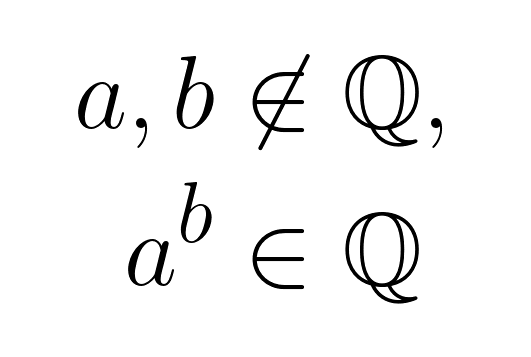The Python 🐍 problem-solving bootcamp 🚀 is starting soon. Join the second cohort now!

Let's prove that there are two irrational numbers, call them $$a$$ and $$b$$, such that $$a^b$$ is a rational number! And let's do it in a tweet.